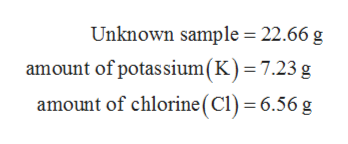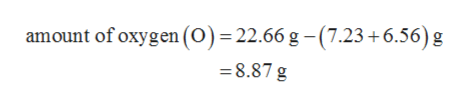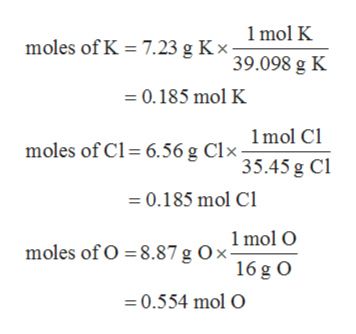A 22.6622.66 g sample of a compound contains 7.237.23 g of potassium, K,K, 6.566.56 g of chlorine, Cl,Cl, and oxygen, O.O. Calculate the empirical formula.Insert subscripts as needed.

Question

A 22.6622.66 g sample of a compound contains 7.237.23 g of potassium, K,K, 6.566.56 g of chlorine, Cl,Cl, and oxygen, O.O. Calculate the empirical formula.

Insert subscripts as needed.

Step 1

The values are given as follows:help_outlineImage TranscriptioncloseUnknown sample 22.66 g amount of potassium(K)= 7.23 g amount of chlorine (CI) 6.56 g fullscreen
Step 2

The amount of oxygen (O) in the unknown sample can be evaluate as follows:help_outlineImage Transcriptioncloseamount of oxygen (0) = 22.66 g -(7.23 +6.56) g 8.87 g fullscreen
Step 3

The mole ratios of each element are determined by dividing each element mass with ...help_outlineImage Transcriptionclose1 mol K 7.23 g K. x 39.098 g K moles of K 0.185 mol K 1mol Cl moles of Cl 6.56 g C 35.45 g Ci 0.185 mol Cl 1 mol O ох moles of O 8.87 g O 16 g O 0.554 mol O fullscreen

Want to see the full answer?

See Solution

Want to see this answer and more?

Our solutions are written by experts, many with advanced degrees, and available 24/7

See Solution
Tagged in

General Chemistry